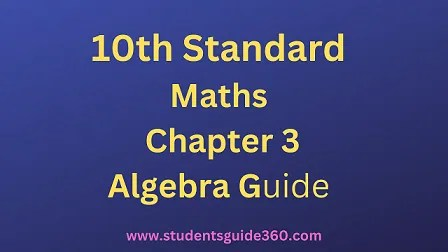# 10th Maths Guide Chapter 3 Algebra Exercise 3.4

10th Standard Maths Chapter 3 Algebra Samacheer Kalvi Guide Exercise 3.4 Book Back Answers Solutions. TN 10th SSLC Samacheer Kalvi Guide. 10th All Subject Guide – Click Here. Class 1 to 12 All Subject Book Back Answers – Click Here## 10th Maths Guide Chapter 3 Algebra Exercise 3.4

### 1. Reduce each of the following rational expressions to its lowest form.Solution:### 2. Find the excluded values, if any of the following expressions.Solution:

(i) yy2−25=y(y+5)(y−5) is undefined when (y + 5) (y – 5) = 0 that is y = -5, 5
∴ The excluded values are -5, 5

(ii) tt2−5t+6 is undefined when t2 – 5t + 6 = 0 i.e.
(t – 3) (t – 2) = 0 ⇒ t = 3, 2
∴ The excluded values are 3, 2

(iii) x2+6x+8×2+x−2 is undefined when x2 + x – 2 = 0 i.e.
(x + 2) (x + 1) = 0
∴ The excluded values are 2, 1

(iv) x3−27×3+x2−6x is undefined when x3 + x2 – 6x = 0, i.e
x(x2 + x – 6) = 0
x(x + 3) (x – 2) = 0
∴ The excluded values are -3, 2